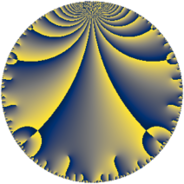# Properties

 Label 165.6.aLevel $165$ Weight $6$ Character orbit 165.a Rep. character $\chi_{165}(1,\cdot)$ Character field $\Q$ Dimension $32$ Newform subspaces $8$ Sturm bound $144$ Trace bound $4$

# Related objects

## Defining parameters

 Level: $$N$$ $$=$$ $$165 = 3 \cdot 5 \cdot 11$$ Weight: $$k$$ $$=$$ $$6$$ Character orbit: $$[\chi]$$ $$=$$ 165.a (trivial) Character field: $$\Q$$ Newform subspaces: $$8$$ Sturm bound: $$144$$ Trace bound: $$4$$ Distinguishing $$T_p$$: $$2$$

## Dimensions

The following table gives the dimensions of various subspaces of $$M_{6}(\Gamma_0(165))$$.

Total New Old
Modular forms 124 32 92
Cusp forms 116 32 84
Eisenstein series 8 0 8

The following table gives the dimensions of the cuspidal new subspaces with specified eigenvalues for the Atkin-Lehner operators and the Fricke involution.

$$3$$$$5$$$$11$$FrickeDim.
$$+$$$$+$$$$+$$$$+$$$$5$$
$$+$$$$+$$$$-$$$$-$$$$3$$
$$+$$$$-$$$$+$$$$-$$$$3$$
$$+$$$$-$$$$-$$$$+$$$$3$$
$$-$$$$+$$$$+$$$$-$$$$5$$
$$-$$$$+$$$$-$$$$+$$$$3$$
$$-$$$$-$$$$+$$$$+$$$$3$$
$$-$$$$-$$$$-$$$$-$$$$7$$
Plus space$$+$$$$14$$
Minus space$$-$$$$18$$

## Trace form

 $$32 q + 36 q^{3} + 468 q^{4} - 180 q^{6} + 72 q^{7} + 2592 q^{9} + O(q^{10})$$ $$32 q + 36 q^{3} + 468 q^{4} - 180 q^{6} + 72 q^{7} + 2592 q^{9} - 100 q^{10} + 1728 q^{12} + 1024 q^{13} + 1240 q^{14} + 900 q^{15} + 6220 q^{16} + 1912 q^{17} + 1752 q^{19} - 3800 q^{20} - 4608 q^{21} + 1936 q^{22} - 2416 q^{23} - 540 q^{24} + 20000 q^{25} + 15472 q^{26} + 2916 q^{27} + 12936 q^{28} - 2128 q^{29} - 11488 q^{31} - 30040 q^{32} + 4356 q^{33} + 19792 q^{34} - 6200 q^{35} + 37908 q^{36} + 20032 q^{37} - 67624 q^{38} + 32112 q^{39} - 6300 q^{40} - 3584 q^{41} + 2304 q^{42} + 19880 q^{43} + 4840 q^{44} - 101872 q^{46} - 5792 q^{47} + 64512 q^{48} + 80864 q^{49} - 15336 q^{51} + 54400 q^{52} - 77312 q^{53} - 14580 q^{54} + 24200 q^{55} + 130344 q^{56} - 64440 q^{57} + 33384 q^{58} + 53888 q^{59} + 83700 q^{60} + 119104 q^{61} + 107384 q^{62} + 5832 q^{63} + 151156 q^{64} - 200 q^{65} + 2000 q^{67} - 88496 q^{68} - 32976 q^{69} + 105400 q^{70} - 22368 q^{71} - 192944 q^{73} - 15184 q^{74} + 22500 q^{75} - 60640 q^{76} - 59048 q^{77} - 61344 q^{78} + 76712 q^{79} - 88800 q^{80} + 209952 q^{81} - 107128 q^{82} - 150568 q^{83} - 91008 q^{84} - 128600 q^{85} - 462912 q^{86} - 64584 q^{87} + 92928 q^{88} + 254480 q^{89} - 8100 q^{90} - 125664 q^{91} - 93416 q^{92} + 354528 q^{93} + 269840 q^{94} - 111200 q^{95} + 9540 q^{96} - 113312 q^{97} - 5032 q^{98} + O(q^{100})$$

## Decomposition of $$S_{6}^{\mathrm{new}}(\Gamma_0(165))$$ into newform subspaces

Label Dim $A$ Field CM Traces A-L signs $q$-expansion
$a_{2}$ $a_{3}$ $a_{5}$ $a_{7}$ 3 5 11
165.6.a.a $3$ $26.463$ 3.3.34253.1 None $$-7$$ $$27$$ $$75$$ $$-172$$ $-$ $-$ $+$ $$q+(-2-\beta _{1})q^{2}+9q^{3}+(7+4\beta _{1}+\beta _{2})q^{4}+\cdots$$
165.6.a.b $3$ $26.463$ 3.3.3368.1 None $$-2$$ $$27$$ $$-75$$ $$-232$$ $-$ $+$ $-$ $$q+(-1-\beta _{1})q^{2}+9q^{3}+(8+4\beta _{2})q^{4}+\cdots$$
165.6.a.c $3$ $26.463$ 3.3.18257.1 None $$2$$ $$-27$$ $$75$$ $$-68$$ $+$ $-$ $-$ $$q+(1-\beta _{1})q^{2}-9q^{3}+(-14-\beta _{1}+\beta _{2})q^{4}+\cdots$$
165.6.a.d $3$ $26.463$ 3.3.788.1 None $$2$$ $$-27$$ $$75$$ $$152$$ $+$ $-$ $+$ $$q+(1+\beta _{2})q^{2}-9q^{3}+(-8-4\beta _{1})q^{4}+\cdots$$
165.6.a.e $3$ $26.463$ 3.3.307532.1 None $$7$$ $$-27$$ $$-75$$ $$92$$ $+$ $+$ $-$ $$q+(2+\beta _{1})q^{2}-9q^{3}+(24+2\beta _{1}+\beta _{2})q^{4}+\cdots$$
165.6.a.f $5$ $26.463$ $$\mathbb{Q}[x]/(x^{5} - \cdots)$$ None $$-2$$ $$45$$ $$-125$$ $$184$$ $-$ $+$ $+$ $$q-\beta _{1}q^{2}+9q^{3}+(2^{4}+\beta _{2})q^{4}-5^{2}q^{5}+\cdots$$
165.6.a.g $5$ $26.463$ $$\mathbb{Q}[x]/(x^{5} - \cdots)$$ None $$-1$$ $$-45$$ $$-125$$ $$116$$ $+$ $+$ $+$ $$q-\beta _{1}q^{2}-9q^{3}+(5^{2}+\beta _{1}+\beta _{3})q^{4}+\cdots$$
165.6.a.h $7$ $26.463$ $$\mathbb{Q}[x]/(x^{7} - \cdots)$$ None $$1$$ $$63$$ $$175$$ $$0$$ $-$ $-$ $-$ $$q+\beta _{1}q^{2}+9q^{3}+(28+\beta _{2})q^{4}+5^{2}q^{5}+\cdots$$

## Decomposition of $$S_{6}^{\mathrm{old}}(\Gamma_0(165))$$ into lower level spaces

$$S_{6}^{\mathrm{old}}(\Gamma_0(165)) \cong$$ $$S_{6}^{\mathrm{new}}(\Gamma_0(3))$$$$^{\oplus 4}$$$$\oplus$$$$S_{6}^{\mathrm{new}}(\Gamma_0(5))$$$$^{\oplus 4}$$$$\oplus$$$$S_{6}^{\mathrm{new}}(\Gamma_0(11))$$$$^{\oplus 4}$$$$\oplus$$$$S_{6}^{\mathrm{new}}(\Gamma_0(15))$$$$^{\oplus 2}$$$$\oplus$$$$S_{6}^{\mathrm{new}}(\Gamma_0(33))$$$$^{\oplus 2}$$$$\oplus$$$$S_{6}^{\mathrm{new}}(\Gamma_0(55))$$$$^{\oplus 2}$$